Next: Decay of the molecular Up: The standard Vesman model Previous: Formulation of the formation

### Wave functions

The initial and final wave functionsin the molecular formation matrix element caculation are usually approximated as:(43)

whereis the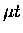ground state wave function withbeing the interparticle vector from t to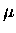, andis the initial D2 wave function with internuclear vectorand electron vectors. The factordescribes the relative motion of the centre of mass of theand D2.

The final wave function is written:(44)

whereis the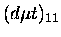wave function, andis the final state molecular complex wave function where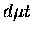is replaced by a fictitious particle of mass and charge equal to the sum ofand located at the centre of mass of.

Following Menshikov , most authors use an analytical approximation ofwith its asymptotic form valid in the limit of infinite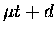separation, since the main contribution to the matrix element integral comes from the region where theseparation is large. The asymptotic wave function is characterized by a normalization constant C [149,150]. Recently Kino et al.  obtained a new value of C using Kamimura's variational wave function (Section 2.1.4, page), calculated with the Gaussian basis functions in the Jacobi co-ordinate. As we have discussed, Kamimura's wave function treats the break up channel intodirectly, hence it gives an accurate description of asymptotic behaviour, while overcoming the difficulty of the Gaussian basis (fast dumping'') by using a sufficiently large number of basis functions. The formation rates are proportional to the square of the constant C, and its new value decreased the predicted formation rates by 14% compared to an earlier calculation in Ref.  using the Adiabatic Representation, but increased them by 33% compared to Ref.  using a variational wave function with the Slater-type basis functions . It is interesting to note that the Slater-type basis method, which was used so successfully in the energy level calculations (see Table 2.1), gives a less accurate description (assuming Kino et al. are correct) of the asymptotic wave function compared even to the Adiabatic Representation method, perhaps illustrating the difficulty in achieving a unified description of the non-adiabatic three body problem. In our analysis, the new value of C by Kino et al. is used.Next: Decay of the molecular Up: The standard Vesman model Previous: Formulation of the formation